# Notes on stochastic count processes with independent non stationary increments

## Problem Statement

Letthus, we construct a stochastic process as, this kind of random variable are called hypoexponential random variables. We define a counting process such that. This allows to model a certain relation between events, for example to have a shorter expected waiting time for a second event if the first event was observed.

Statement 1:is no Poisson process.
To see this let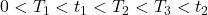andthenwhile. Since the density ofis given by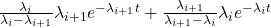withthe process is non stationary and can thus not be a Poisson process.

Statement 2: The (hypoexponential) distribution ofis given byfor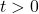andelse where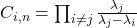and thus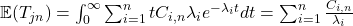.

(1)## Estimation

To estimatewe construct a different process. For fixed, letbe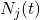sorted descending by size.this is the amount of all processes where the-th event was reached at time. Let, hereis determined by the ordering of, this is also often referred to as exposure.is now estimated with.

The sum of order statistics exponential random variables is given bythus the joined distribution is given by

(2)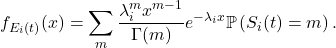Note thatand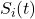are not independent, in particular

(3)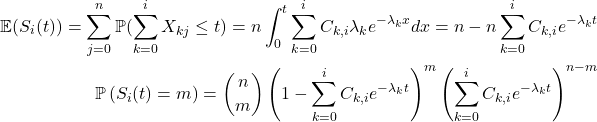(see Statement 2), if the realisation ofis relatively small than for giventhe probability that the event was observed is higher. For simplicity, in the following, we will consider a special case where, thenand the sum of exponential random variables is given by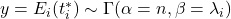, thus

(4)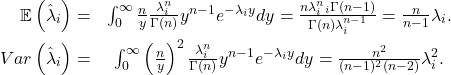Therefore the estimatoris unbiased while the MSE is given by. Which reflects the well-known dichotomy between unbiasedness and minimal MSE.

For the general case

(5)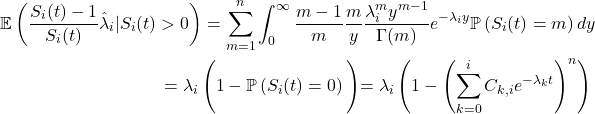thus we face a slight bias, the cause of which is when there are too few observations available.H. N. Nagaraja, “Order statistics from independent exponential random variables and the sum of the top order statistics,” in Advances in distribution theory, order statistics, and inference, N. Balakrishnan, J. M. Sarabia, and E. Castillo, Eds., Boston, MA: Birkhäuser boston, 2006, p. 173–185.
[Bibtex]
@Inbook{Nagaraja2006,
author="Nagaraja, H. N.",
editor="Balakrishnan, N.
and Sarabia, Jos{\'e} Mar{\'i}a
and Castillo, Enrique",
title="Order Statistics from Independent Exponential Random Variables and the Sum of the Top Order Statistics",
bookTitle="Advances in Distribution Theory, Order Statistics, and Inference",
year="2006",
publisher="Birkh{\"a}user Boston",
abstract="Let X(1)<...

 Posted in Introduction 
 Leave a Reply Your email address will not be published. Required fields are marked *Comment * Name * Email * Website Δdocument.getElementById( "ak_js_1" ).setAttribute( "value", ( new Date() ).getTime() ); This site uses Akismet to reduce spam. Learn how your comment data is processed.